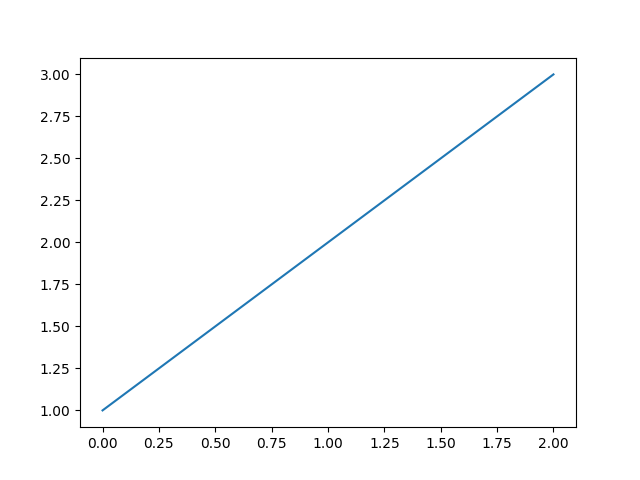Agg Buffer¶

Use backend agg to access the figure canvas as an RGBA buffer, convert it to an array, and pass it to Pillow for rendering.import numpy as np

from matplotlib.backends.backend_agg import FigureCanvasAgg
import matplotlib.pyplot as plt

plt.plot([1, 2, 3])

canvas = plt.gcf().canvas

agg = canvas.switch_backends(FigureCanvasAgg)
agg.draw()
X = np.asarray(agg.buffer_rgba())

# Pass off to PIL.
from PIL import Image
im = Image.fromarray(X)

# Uncomment this line to display the image using ImageMagick's display tool.
# im.show()

Keywords: matplotlib code example, codex, python plot, pyplot Gallery generated by Sphinx-Gallery